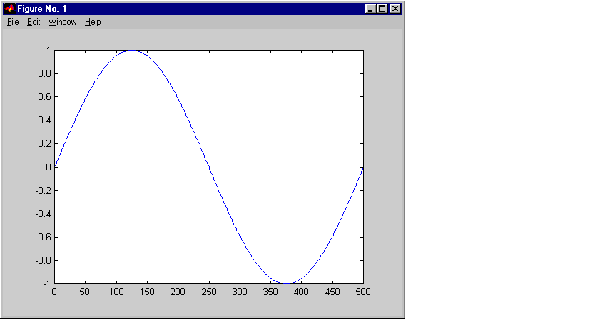External InterfacesCalling Functions from C MEX-Files

It is possible to call MATLAB functions, operators, M-files, and other MEX-files from within your C source code by using the API function `mexCallMATLAB`. This example creates an `mxArray`, passes various pointers to a subfunction to acquire data, and calls `mexCallMATLAB` to calculate the sine function and plot the results.

• ```/*
* =============================================================
* sincall.c
*
* Example for illustrating how to use mexCallMATLAB
*
* Creates an mxArray and passes its associated pointers (in
* this demo, only pointer to its real part, pointer to number of
* rows, pointer to number of columns) to subfunction fill() to
* get data filled up, then calls mexCallMATLAB to calculate sin
* function and plot the result.
*
* This is a MEX-file for MATLAB.
* Copyright (c) 1984-2000 The MathWorks, Inc.
* =============================================================
*/

/* \$Revision: 1.4 \$ */

#include "mex.h"
#define MAX 1000

/* Subroutine for filling up data */
void fill(double *pr, int *pm, int *pn, int max)
{
int i;

/* You can fill up to max elements, so (*pr) <= max. */
*pm = max/2;
*pn = 1;
for (i = 0; i < (*pm); i++)
pr[i] = i * (4*3.14159/max);
}

/* The gateway routine */
void mexFunction(int nlhs, mxArray *plhs[],
int nrhs, const mxArray *prhs[])
{
int     m, n, max = MAX;
mxArray *rhs, *lhs;

rhs = mxCreateDoubleMatrix(max, 1, mxREAL);

/* Pass the pointers and let fill() fill up data. */
fill(mxGetPr(rhs), &m, &n, MAX);
mxSetM(rhs, m);
mxSetN(rhs, n);

/* Get the sin wave and plot it. */
mexCallMATLAB(1, lhs, 1, rhs, "sin");
mexCallMATLAB(0, NULL, 1, lhs, "plot");

/* Clean up allocated memory. */
mxDestroyArray(rhs);
mxDestroyArray(lhs);

return;
}
```

Running this example

• ```sincall
```

displays the resultsNote    It is possible to generate an object of type mxUNKNOWN_CLASS using `mexCallMATLAB`. See the example below.

The following example creates an M-file that returns two variables but only assigns one of them a value.

• ```function [a,b] = foo[c]
a = 2*c;
```

MATLAB displays the following warning message.

• ```Warning: One or more output arguments not assigned during call to
'foo'.
```

If you then call `foo` using `mexCallMATLAB`, the unassigned output variable will now be of type `mxUNKNOWN_CLASS`.Handling Sparse Arrays Advanced Topics© 1994-2005 The MathWorks, Inc.